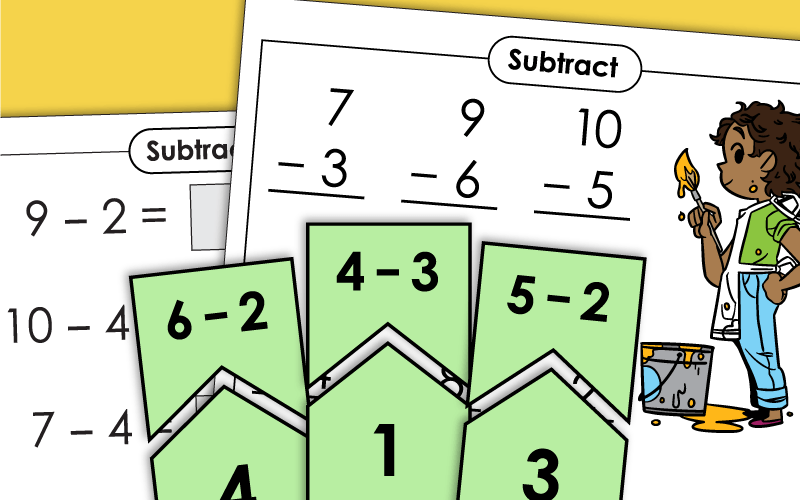# Basic Subtraction (Within 10)

This page has basic subtraction worksheets, activities. All problems have minuends and quotients of 10 or less. These are written for kindergarten-level students.

For basic subtractions with minuends to 20 and quotients to 10, please see our main basic subtraction page.## Basic Subtraction Games

In this math card game, students take turns matching each subtraction fact with its answer.
Print out a subtraction bingo board for each student in your class. The teacher calls out subtraction facts and the class marks the answers on their boards.
This is a fun, single-player game to play with dice. Your student will roll two dice and write the numbers in the boxes (larger number first) and solve the subtraction problem.
Players take turns rolling a pair of dice. After each roll, the player subtracts the smaller number from the larger number. The player with the greatest difference wins the round. All minuends and answers are 10 or less.
Students roll a die and match the number rolled with a column on the subtraction chart. They read and answer all of the subtraction facts in the column as accurately and quickly as possible. All minuends and answers are 10 or less. (ex: 7-3=4)

## Concept Worksheets

This printable worksheet has 4 word problems with a picture model to help students solve the subtraction problem.
Use the pictures of counters to help you solve the basic subtraction problems.
Solve the basic subtraction problems by drawing counters in the space provided and circling the counters to make up the minuend.
On this worksheet, students will determine how many counters are missing from the problem and draw them in to complete the subtraction problem.
This worksheet provides a space for students to draw pictures to help them solve the subtraction problems.
Using pictures of fruit as a visual aid, student will solve these four basic subtraction problems.

## Cut-and-Glue Worksheets

Students will find the difference for each of these problems. Use scissors and glue to match the numbers to the correct boxes.
Cut apart the puzzle pieces. Match subtraction problems with their answers. Make a great learning center. (Note: There is an alternate, full-color version of this activity. If you'd like the color version, click the "alt" button below.)
Students can practice basic subtraction with this printable puzzle activity.

## Number LineSubtraction

Have your students figure out which subtraction problems are being shown on each of the number lines.
These printable number line worksheets make provide a visual aid to help students identify the subtrahend in each subtraction fact.
Students will "count back" on the number lines to find the missing subtrahend in each subtraction fact.
This worksheet has number lines to help students solve the subtraction facts.
Students will use the number line to determine the addition or subtraction problem and write it on the line.

## Subtraction Fact Practice

Write the answers to the subtraction facts on the caterpillar. All facts have minuends and quotients of 10 or less. (example: 7-3)
Tell which facts are written correctly and which ones are incorrect. When a fact is incorrect, cross off the difference and write the correct answer.

## Mystery Pictures

Find the differences for these subtraction facts, then color according to the key.
Your students will have fun solving the subtraction problems and coloring in the mystery picture to reveal school supplies on a desk.
On this worksheet, students will solve basic subtraction problems with differences of 10 or less. The finished picture will reveal a read bird sitting on its nest.
After writing the differences for the problems on the page, color the sea monster (dragon) according to the color code at the bottom of the picture.
Have your students solve these very basic subtraction problems. Then they will use the color key to color in the puzzle to reveal sea shells on a beach.
After students find the answers to the subtraction facts, they can color each space according to the key to reveal a gray and blue whale.

## Basic Subtraction Worksheet Generator

Basic Subtraction Worksheet Generator

Use this generator to make your own custom subtraction worksheets with basic facts. You choose the range for the subtrahends and the differences. This tool lets you to create worksheets with 25 or 50 problems.

## Subtraction Fact Practice

Students can solve nine subtraction problems on this printable worksheet. Minuends are 10 or less. Images on this worksheet are of kids playing winter sports.
Practice solving basic subtraction problems with minuends of 10 or less with this printable. These 9 math facts are in an easy-to-read font for early learners.
This printable has 9 basic subtraction facts on it. Minuends are 10 or less (ex. 10-7=3, 9-3=6). This worksheet includes images of a mouse and his cheese.
Challenge your students to solve these basic subtraction facts on this printable worksheet. Minuends are all 10 or less.
These basic subtraction problems all have minuends of 10 or less. There are nine facts to solve which are in a large font for early learners.
Have your students practice solving subtraction facts horizontally with this worksheet. There are 12 subtraction facts to solve with minuends of 10 or less.

## Speed Drill Worksheets

Challenge your students to write the answers to all fifty subtraction facts as quickly as possible.
Here's an alternate version of the drill sheet above.
One more version of our speed subtraction drill. This timed quiz has 50 questions.

## Flashcards

Download and cut apart subtraction flashcards. Use them for small group instruction, independent practice, or classroom games.
More Subtraction Worksheets

This page lists the units for all of the more advanced subtraction worksheets. Includes basic subtraction with minuends up to 20, fact families, and multi-digit subtraction.

These worksheets combine both basic subtraction and addition math problems.

This page has fact family =and number bond worksheets to demonstrate the relationship between subtraction and addition.